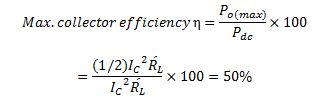# Maximum Collector Efficiency of Transformer Coupled Class A Power Amplifier

## Maximum Collector Efficiency of Transformer Coupled Class A Power Amplifier

In class A amplifier , the load can be either connected directly in the collector or it can be transformer coupled .

The latter method is often preferred for two main reasons. First, transformer coupling permits impedance matching and secondly it keeps the d.c. power loss small because of the small resistance of the transformer primary winding .

Fig.1 shows the transformer coupled class A power amplifier .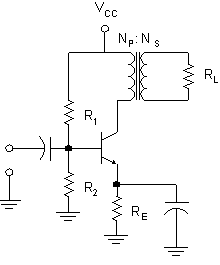Fig.1 : Transformer coupled class A amplifier

In order to determine maximum collector efficiency, we will refer to the output characteristics shown in fig.2 .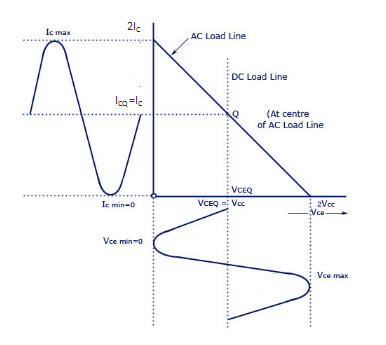Fig. 2

Under zero signal condition, the effective resistance in the collector circuit is that of the primary winding of the transformer. The primary resistance has a very small value and is assumed zero .

Therefore, d.c. load line is a vertical line rising from VCC   as shown in fig.2 .

When signal is applied, the collector current will vary about the operating point Q .

In order to get maximum a.c. power output (and hence maximum collector η ), the peak value of collector current due to signal alone should be equal to the zero signal collector current IC .

In terms of a.c. load line , the operating point Q should be located at the centre of a.c. load line .

### Derivation of Maximum Collector Efficiency

During the peak of the positive half-cycle of the signal, the total collector current is 2 IC  and vce = 0 .

During the negative peak of the signal, the collector current is zero and vce = 2 VCC

So, Peak-to peak collector – emitter voltage is :Peak-to-peak collector current is :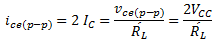Where R′L  is the reflected value of load RL  and appears in the primary of the transformer .

If n= (Np/N) is the turn ratio of the transformer, then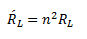d.c. power output,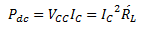Max. a.c. output power,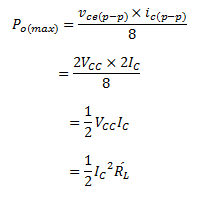Hence,